# Counting Based on Multiple Criteria

Last week we talked about the COUNTIF formula and how you could use it to count rows based on a single criterion.

But what if you wanted to count rows based on multiple criteria? If you haven’t guessed already, the answer is with COUNTIFS!

## COUNTIFS

COUNTIFS is a function that counts data based on multiple criteria from multiple data ranges.

COUNTIFS is structurally similar to COUNTIF, but it’s so much more powerful. Let’s use the same data set from the COUNTIF post and expand on it. Download the example to follow.

Here we have about 140 rows of hypothetical spending data, from the months of October through December.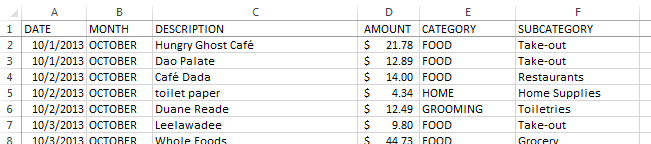In columns H & I, we used COUNTIF to count the number of times we’ve spent during each month.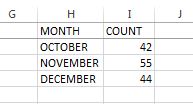Now let’s use COUNTIFS to see how many times we’ve spent in specific categories within each month. First enter your months and categories in columns K and L.In cell M2, type “=COUNTIFS(B2:B142,”OCTOBER”,E2:E142,”CLOTHING”).” This formula is telling Excel to look in the range B2:B142 and count all the instances of “OCTOBER,” and look in the range E2:E142 and count all the instances of “CLOTHING.” Excel will only count the rows where both criteria are met.Alternatively, instead of typing out the criteria “OCTOBER” and “CLOTHING,” you could select the cell references K2 and L2 respectively. Since we will want to copy this formula down to the bottom of the section, let’s also make the data range rows absolute. Here’s what the formula should look like now.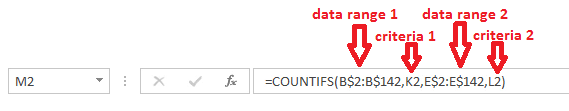It seems that we only shopped for clothing once in October.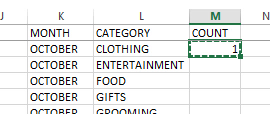Fill the formula all the way down to see how many times we spent on each category for each month.

You could even add another criteria to the mix! Let’s use COUNTIFS to see how many times we’ve spent in each subcategory, by category, by month.

In columns O-Q, enter all your months, categories, and subcategories. We’ll put in our COUNTIFS formula in column R.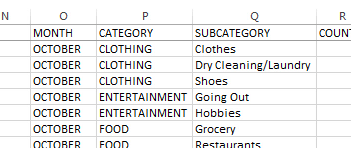In R2, enter “COUNTIFS(B\$2:B\$142,O2,E\$2:E\$142,P2,F\$2:F\$142,Q2).”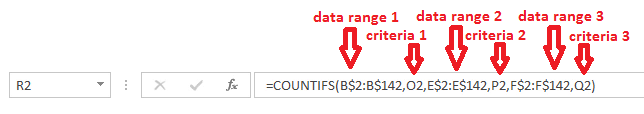Then fill the formula all the way down.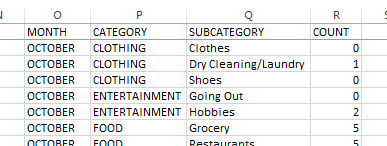Neat, right? If you wanted to, you could keep going, adding more and more criteria to your COUNTIFS. It’s an easy formulaic way of counting based on a bunch of criteria!